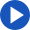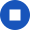## equate

Regular
Change speakerYou're listening to Joanna
She has an American accent and expects you to use American spelling.

•••••Past

• I equated
• you equated
• he/she/it equated
• we equated
• you equated
• they equated

Present

• I equate
• you equate
• he/she/it equates
• we equate
• you equate
• they equate

Future

• I will equate
• you will equate
• he/she/it will equate
• we will equate
• you will equate
• they will equate

JoannaYouReset